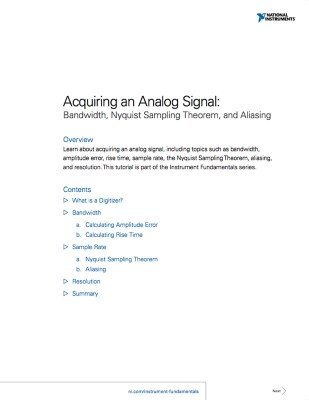# Acquiring an Analog Signal: Bandwidth, Nyquist Sampling Theorem, and Aliasing

## Overview

Learn about acquiring an analog signal, including topics such as bandwidth, amplitude error, rise time, sample rate, the Nyquist Sampling Theorem, aliasing, and resolution.Topics Covered What is a DigitizerBandwidth: corner frequencies, calculating amplitude error, calculating rise timeSample rate: Nyquist sampling theorem, aliasingResolution and precision
Next Steps